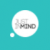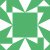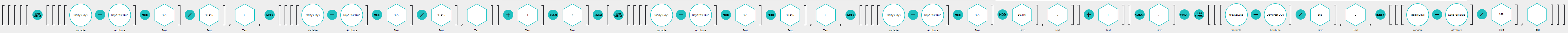# Math on Dates

## Is it possible to do math on dates in Justinmind Prototyper? I'm building a prototype that contains "start date" and "end date" fields, and I want the start date to be today, and the end date to be a year from now. Filling in the start date with today's date is pretty easy on page load. I don't see any way to do math on a date though, and I just want to verify if that's possible?

Replies (5)I'm afraid the only thing you can do is to compare two dates. 'Summing' dates is not supported right now.I've been thinking and maybe you can try to do something like this: http://www.justinmind.com/usernote/te...Gotcha, that will work in a pinch. Thanks!So I tried to use this technique to do math on dates and though I solved it, it was DAMN HARD!!! In order to use this technique you must use a Math.Floor and Math.Ceil functions. Since I didn't have those I used the substring routine to strip off numbers past the decimal point, which works fine so long as your calculations HAVE a decimal point. Essentially here's how I solved it:

For this example, tD = todaysDays (i.e. number of days to today) and sD = subtracted days because I was calculating days in the past from today

1.) Calculate number of days to today: todaysDays(tD) = (month-1*avg number of days in a month)+day+(year*days in a year)

tD should be Math.Floor((substr(systemDate,0,2)*30.416)+(substr(systemDate,3,5)+(substr(systemDate,6,11)*'365'))) but instead we round it:

tD = round((((substr(systemDate,0,2)-'1')*'30.416')+(substr(systemDate,3,5)+(substr(systemDate,6,11)*'365')),'0')

2. Structure the data: (MonthCalc) concat "/" concat (DateCalc) concat "/" concat (YearCalc)

3. Calculate MonthCalc: Math.Floor( ( ( todaysDate - subtractDate modulus daysInAYear ) / avgDaysPerMonth )) + 1 (all month math performed zero based and added 1 to make it read correctly)

MonthCalc = (substr((((tD-sD) mod '356') / '30.416'), 0, index((((tD-sD) mod '365') / '30.416' ), '.')) + 1)

4. Calculate DateCalc: DateCalc = Math.Floor ( ( ( ( todaysDate - subtractDate )modulus daysInAYear ) modulus avgDaysPerMonth)) + 1 (compensation for lack of Math.Ceil function to round us up)

DateCalc = (substr((((tD-sD)mod '365')mod '30.416'), 0, index((((tD-sD)mod '365')mod '30.416'),'.'))+1)

5. Calculate YearCalc: YearCalc = Math.Floor( ( todaysDate - subtractDate) / daysInAYear)

YearCalc = substr(((tD-sD)/365),0,index(((tD-sD)/365),'.')))

If you put it all together it looks like the attached screenshot (note my variable name for subtractDate is DaysPastDue) I tried to include it here but it's microscopic as you can see. That's all of the nodes needed to calculate 1 date subtraction. Can we maybe have a better way? PLEASE????Hi Nate,

Not sure if you were posting your response for those who continue to search for a solution to this problem, as the OP is five years old, but there is a date arithmetic prototype posted in response to a question from about 3 months ago here:

Hope you find it useful. Cheers!Yes, I did post it to help others who might be looking for this answer. It took me hours to come up with a solution that was close enough to past muster. I took the approach I did because I wanted to update a data master on page load and needed the entire formula in one object attribute so it'd update each of the data objects.

I agree, that a truncate function, math.floor, and math.ceil functions would really help with these calculations.

The example provided in the other post required 35 variables and over 45 actions and 22 conditional statements to perform a simple date comparison. IMO that's pretty extreme to do something so common. We should make it easier for users of Justinmind to do this. Date comparisons and calculations are critical for many different prototype scenarios. I could have used it in at least a dozen different prototypes if it had existed and wasn't such a pain to do.

Thanks for sharing though."We should make it easier for users of Justinmind to do this. Date comparisons and calculations are critical for many different prototype scenarios."

Agreed!